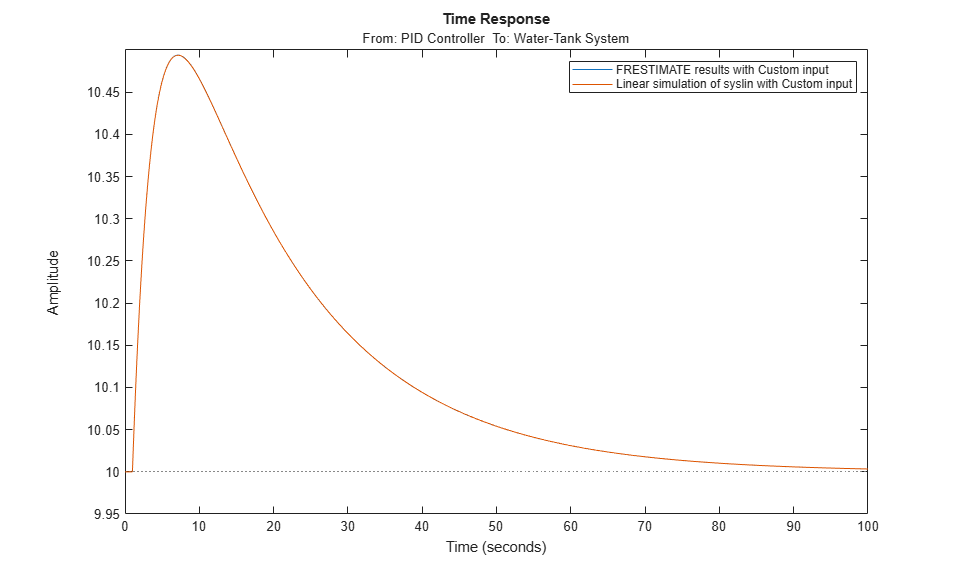frest.simCompare

Package: frest

Plot time-domain simulation of nonlinear and linear models

Syntax

frest.simCompare(simout,sys,input)
frest.simCompare(simout,sys,input,x0)
[y,t] = frest.simCompare(simout,sys,input)
[y,t,x] = frest.simCompare(simout,sys,input,x0)

Description

frest.simCompare(simout,sys,input) plots both

• Simulation output, simout, of the nonlinear Simulink model

You obtain the output from the frestimate command.

• Simulation output of the linear model sys for the input signal input

The linear simulation results are offset by the initial output values in the simout data.

frest.simCompare(simout,sys,input,x0) plots the frequency response simulation output and the simulation output of the linear model with initial state x0. Because you specify the initial state, the linear simulation result is not offset by the initial output values in the simout data.

[y,t] = frest.simCompare(simout,sys,input) returns the linear simulation output response y and the time vector t for the linear model sys with the input signal input. This syntax does not display a plot. The matrix y has as many rows as time samples (length(t)) and as many columns as system outputs.

[y,t,x] = frest.simCompare(simout,sys,input,x0) also returns the state trajectory x for the linear state space model sys with initial state x0.

Examples

collapse all

frest.simCompare lets you lets you examine the results of frequency response estimation in the time domain. You can compare the simulated model response to the response of a linear model of the system, such as one obtained by exact linearization.

Estimate the closed-loop response of the plant in the watertank model. First, open the model.

model = 'watertank';
open_system(model)Define a linearization I/O set that specifies the plant, and find a steady-state operating point for estimation.

io(1)=linio('watertank/PID Controller',1,'input');
io(2)=linio('watertank/Water-Tank System',1,'output');

watertank_spec = operspec(model);
opOpts = findopOptions('DisplayReport','off');
op = findop(model,watertank_spec,opOpts);

Create an input signal for estimation. For this example, use a step input.

input = frest.createStep('FinalTime',100);

Estimate the frequency response of the specified portion of the model, using the simout output argument to store the data generated during the estimation process. Also, linearize the model using the same I/O set and operating point.

[sysest,simout] = frestimate(model,op,io,input);
syslin = linearize(model,io,op);

Examine the time-domain responses of the linearized model and the Simulink model to the same input signal.

frest.simCompare(simout,syslin,input)
legendIn this example, the responses are virtually identical.